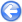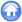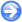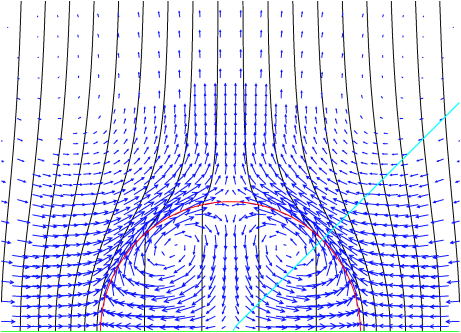### 14.4PASS: Equilibrium of a droplet suspended in an electric field

Author
Jose M. López-Herrera Sánchez
Command
sh electro.sh
Version
100527
Required files
electro.sh figure.gfv convergence.ref
Running time
14 minutes 34 seconds

A conducting droplet is suspended in a conducting liquid of the same density. A constant horizontal potential difference is imposed far from the droplet. At equilibrium, surface tension balances the normal electrical stresses while recirculations are induced by tangential electrical stresses due to the variable charge distribution along the interface (Figure 209).

 Figure 209: Axisymmetric numerical solution in stationary regime. Equipotential lines (black), interface (red), velocity field (blue) and 45 degrees transect line (light blue).In the limit of Stokes flows, an analytical solution for the velocity field is available [41, 42]. Figure 210 gives a comparison of the numerical and theoretical results for the radial and azimuthal components of the velocity along a transect inclined at 45 degrees.

A more detailed discussion of this simulation is given in .

 Figure 210: Radial and azimuthal components of the velocity along a 45 degrees transect. The lines show the theoretical solution and the symbols the numerical results. The (adaptive) maximum spatial resolution is such that R/h=51.2 on the interface.The total charge is conserved to within round-off errors.